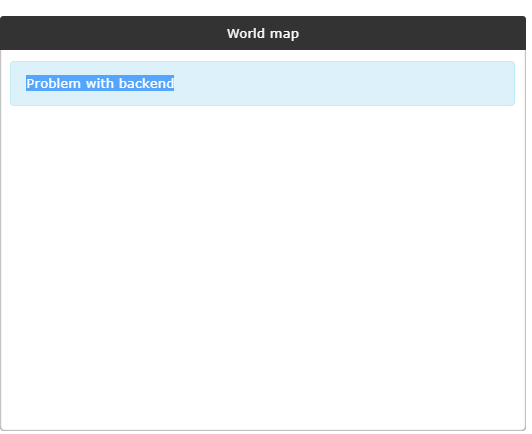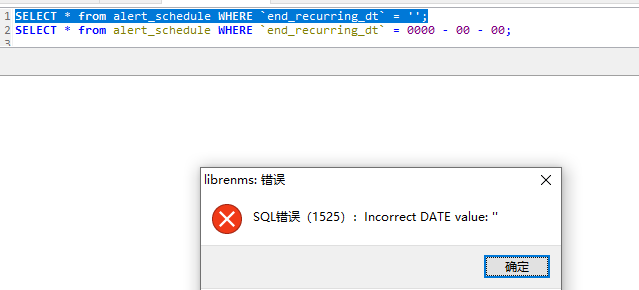# Error after upgrade the mysql to 8.0.19

====================================

Component Version
LibreNMS 1.59-43-g3629db9
DB Schema 2020_01_09_1300_migrate_devices_attribs_table (154)
PHP 7.2.19
MySQL 8.0.19
RRDTool 1.7.2
SNMP NET-SNMP 5.7.2
====================================

hi,all.since we upgraded the mysql to version 8.0.19,we met the problems below:
1、the worldmap is shown as “Problem with backend”and the librenms.log show that an sql error occured:
[2020-01-22 23:19:40] production.ERROR: SQLSTATE[HY000]: General error: 1525 Incorrect DATE value: ‘0000-00-00’ (SQL: select exists(select * from `alert_schedule` inner join `alert_schedulables` on `alert_schedule`.`schedule_id` = `alert_schedulables`.`schedule_id` where ((`recurring` = 0 and `start` <= NOW() and `end` >= NOW()) or (`recurring` = 1 and (`start_recurring_dt` <= date_format(NOW(), ‘%Y-%m-%d’) and (`end_recurring_dt` >= date_format(NOW(), ‘%Y-%m-%d’) or `end_recurring_dt` is null or `end_recurring_dt` = 0000-00-00 or `end_recurring_dt` = )) and `start_recurring_hr` <= date_format(NOW(), ‘%H:%i:%s’) and `end_recurring_hr` >= date_format(NOW(), ‘%H:%i:%s’) and (`recurring_day` like CONCAT(‘%’, date_format(NOW(), ‘%w’), ‘%’) or `recurring_day` is null or `recurring_day` = ))) and ((`alert_schedulable_type` = device and `alert_schedulable_id` = 89) or (`alert_schedulable_type` = device_group and `alert_schedulable_id` in (19)))) as `exists`) {“userId”:15,“exception”:“[object] (Illuminate\Database\QueryException(code: HY000): SQLSTATE[HY000]: General error: 1525 Incorrect DATE value: ‘0000-00-00’ (SQL: select exists(select * from `alert_schedule` inner join `alert_schedulables` on `alert_schedule`.`schedule_id` = `alert_schedulables`.`schedule_id` where ((`recurring` = 0 and `start` <= NOW() and `end` >= NOW()) or (`recurring` = 1 and (`start_recurring_dt` <= date_format(NOW(), ‘%Y-%m-%d’) and (`end_recurring_dt` >= date_format(NOW(), ‘%Y-%m-%d’) or `end_recurring_dt` is null or `end_recurring_dt` = 0000-00-00 or `end_recurring_dt` = )) and `start_recurring_hr` <= date_format(NOW(), ‘%H:%i:%s’) and `end_recurring_hr` >= date_format(NOW(), ‘%H:%i:%s’) and (`recurring_day` like CONCAT(‘%’, date_format(NOW(), ‘%w’), ‘%’) or `recurring_day` is null or `recurring_day` = ))) and ((`alert_schedulable_type` = device and `alert_schedulable_id` = 89) or (`alert_schedulable_type` = device_group and `alert_schedulable_id` in (19)))) as `exists`) at /home/LibreNMS/librenms/vendor/laravel/framework/src/Illuminate/Database/Connection.php:664, Doctrine\DBAL\Driver\PDOException(code: HY000): SQLSTATE[HY000]: General error: 1525 Incorrect DATE value: ‘0000-00-00’ at /home/LibreNMS/librenms/vendor/doctrine/dbal/lib/Doctrine/DBAL/Driver/PDOStatement.php:119, PDOException(code: HY000): SQLSTATE[HY000]: General error: 1525 Incorrect DATE value: ‘0000-00-00’ at /home/LibreNMS/librenms/vendor/doctrine/dbal/lib/Doctrine/DBAL/Driver/PDOStatement.php:117)
[2020-01-22 23:19:41] production.ERROR: SQLSTATE[HY000]: General error: 1525 Incorrect DATE value: ‘’ (SQL: select exists(select * from `alert_schedule` inner join `alert_schedulables` on `alert_schedule`.`schedule_id` = `alert_schedulables`.`schedule_id` where ((`recurring` = 0 and `start` <= NOW() and `end` >= NOW()) or (`recurring` = 1 and (`start_recurring_dt` <= date_format(NOW(), ‘%Y-%m-%d’) and (`end_recurring_dt` >= date_format(NOW(), ‘%Y-%m-%d’) or `end_recurring_dt` is null or `end_recurring_dt` = 0000-00-00 or `end_recurring_dt` = )) and `start_recurring_hr` <= date_format(NOW(), ‘%H:%i:%s’) and `end_recurring_hr` >= date_format(NOW(), ‘%H:%i:%s’) and (`recurring_day` like CONCAT(‘%’, date_format(NOW(), ‘%w’), ‘%’) or `recurring_day` is null or `recurring_day` = ))) and ((`alert_schedulable_type` = device and `alert_schedulable_id` = 1890) or (`alert_schedulable_type` = device_group and 0 = 1))) as `exists`) {“exception”:”[object] (Illuminate\Database\QueryException(code: HY000): SQLSTATE[HY000]: General error: 1525 Incorrect DATE value: ‘’ (SQL: select exists(select * from `alert_schedule` inner join `alert_schedulables` on `alert_schedule`.`schedule_id` = `alert_schedulables`.`schedule_id` where ((`recurring` = 0 and `start` <= NOW() and `end` >= NOW()) or (`recurring` = 1 and (`start_recurring_dt` <= date_format(NOW(), ‘%Y-%m-%d’) and (`end_recurring_dt` >= date_format(NOW(), ‘%Y-%m-%d’) or `end_recurring_dt` is null or `end_recurring_dt` = 0000-00-00 or `end_recurring_dt` = )) and `start_recurring_hr` <= date_format(NOW(), ‘%H:%i:%s’) and `end_recurring_hr` >= date_format(NOW(), ‘%H:%i:%s’) and (`recurring_day` like CONCAT(‘%’, date_format(NOW(), ‘%w’), ‘%’) or `recurring_day` is null or `recurring_day` = ))) and ((`alert_schedulable_type` = device and `alert_schedulable_id` = 1890) or (`alert_schedulable_type` = device_group and 0 = 1))) as `exists`) at /home/LibreNMS/librenms/vendor/laravel/framework/src/Illuminate/Database/Connection.php:664, Doctrine\DBAL\Driver\PDOException(code: HY000): SQLSTATE[HY000]: General error: 1525 Incorrect DATE value: ‘’ at /home/LibreNMS/librenms/vendor/doctrine/dbal/lib/Doctrine/DBAL/Driver/PDOStatement.php:119, PDOException(code: HY000): SQLSTATE[HY000]: General error: 1525 Incorrect DATE value: ‘’ at /home/LibreNMS/librenms/vendor/doctrine/dbal/lib/Doctrine/DBAL/Driver/PDOStatement.php:117)

1. the validate.php can’t recognized the sql version as mysql 8 and still reports the old SQL statements to fix:

the problem has still open.
`production.ERROR: SQLSTATE[HY000]: General error: 1525 Incorrect DATE value: ''`
and I made the test in the mysql,the results below
`end_recurring_dt` = 0000 - 00 - 00” or `end_recurring_dt` = NULL runs ok

but `end_recurring_dt` = ‘’ will cause mysql 8.0.*(>15) relied the “ER_WRONG_VALUE” and frontend’s disasterrelated articles:[MySQL 8.0 and wrong dates – lefred blog: tribulations of a MySQL Evangelist](MySQL 8.0 and wrong dates)
so the solution I thought and I wanna to know:
1、removes the sql which the date_format = ‘’ after mysql 8.0.16

2、where can I find the sql below and how to delete the error sql?:

``````SELECT EXISTS (
SELECT *
WHERE (
(
`recurring` = 0
AND `start` <= NOW()
AND `end` >= NOW()
)
OR (
`recurring` = 1
AND (
`start_recurring_dt` <= date_format(NOW(), '%Y-%m-%d')
AND (
`end_recurring_dt` >= date_format(NOW(), '%Y-%m-%d')
OR `end_recurring_dt` IS NULL
OR `end_recurring_dt` = 0000 - 00 - 00
OR `end_recurring_dt` =
)
)
AND `start_recurring_hr` <= date_format(NOW(), '%H:%i:%s')
AND `end_recurring_hr` >= date_format(NOW(), '%H:%i:%s')
AND (
`recurring_day` LIKE CONCAT (
'%'
,date_format(NOW(), '%w')
,'%'
)
OR `recurring_day` IS NULL
OR `recurring_day` =
)
)
)
) AS `exists`
``````

tmp solution: